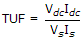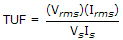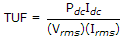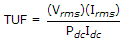# Electronics and Communication Engineering - Power Electronics

Exercise : Power Electronics - Section 8
31.
A fully controlled bridge converter is feeding an RLE load. The load current is I0. The input voltage is Vm sin ωt. The KVL equation to find firing angle a is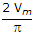cos a = E + I0R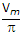cos a = E + I0Rcos a = E - I0Rcos a = E - I0R
Explanation:
No answer description is available. Let's discuss.

32.
A single phase cycloconverter with centre tapped input transformer requires
2 thyristors
4 thyristors
8 thyristors
either 2 or 4 thyristors
Explanation:
No answer description is available. Let's discuss.

33.
The duty cycle of a step down chopper is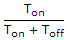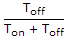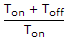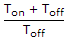Explanation:
No answer description is available. Let's discuss.

34.
In a 3 phase bridge inverter with 120° mode of operation the. number of thyristors conducting at one time are
1
2
3
4